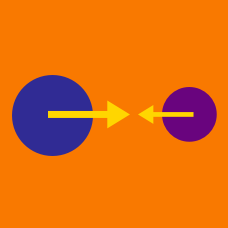Classical Mechanics

# Impulse and the change in momentum

A soccer ball of mass $0.5\text{ kg},$ initially at rest, is kicked by a force of magnitude $1200\text{ N}$ for $15\text{ ms}.$ What is the velocity of the ball after the kick?

A water-rocket of mass $2\text{ kg}$ blasts off from the ground. The lifting force applied on the rocket by the water is $24\text{ N},$ and the air resistance is negligible. Assuming that the mass of the water-rocket does not change, how fast will the rocket be after $5$ seconds?

The gravitational acceleration is $g=10\text{ m/s}^2.$

A man pushes a moving ball on a frictionless floor. If the man pushes with magnitude $11\text{ N}$ for $5$ seconds, what is the change of momentum the ball experiences?

Tiger Woods can hit a golf ball quite hard. A golf ball just after being hit by Mr. Woods has a kinetic energy of $100~\mbox{J}$ and a momentum of $3~\mbox{kg m/s}$. What is the mass of the golf ball in grams?

A golfer strikes a golf ball of mass $0.04\text{ kg}$ with a force of magnitude $400\text{ N}.$ If the time of impact between the golf club and the ball is $3\text{ ms},$ what is the velocity of the ball after the strike?

×